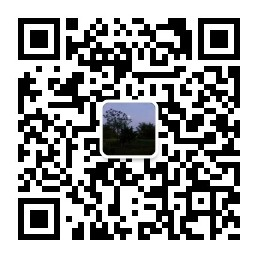# 计算 一个点 附近的地方

### 问题

`select id from tb_location where calc_distance(lng, lat, curLng, curLat) < 2000`

### 优化2 公里范围是一个圆，这个圆的边界是由任意多的点确定的。但一个正方形的边界却是由四个点确定的，所以可以通过把要查找的地点限定在包围这个圆的最小正方形来缩小查找范围。

``````package net.coderbee.gps;

public class GPS {

private final double EARTH_RADIUS = 6378.137; // 地球半径

private static double rad(double d) {
return d * Math.PI / 180.0; // 计算弧长
}

/**
* 计算两个地理坐标之间的距离，
*
* @param Lat1
* @param Lng1
* @param Lat2
* @param Lng2
* @return 距离，单位是米
*/
public double calcDistance(double Lat1, double Lng1, double Lat2,
double Lng2) {
double s = 2 * Math.asin(Math.sqrt(Math.pow(Math.sin(a / 2), 2)
* Math.pow(Math.sin(b / 2), 2)));
s = s * 1000; // 换算成米
return s;

}

/**
* 计算最小正方形的四个经纬度值
*
* @param lng
*            经度
* @param lat
*            纬度
* @param distance
*            距离
* @return
*/
public static double[] getRectangle(double lng, double lat, long distance) {
float delta = 111000;

double minLng = lng - distance
double maxLng = lng + distance
double minLat = lat - (distance / delta);
double maxLat = lat + (distance / delta);
return new double[] { minLng, minLat, maxLng, maxLat };
}
}
``````

``````select id from (
select loc.id, calc_distance(loc.lng,  loc.lat,  :curLng,  :curLat) distance
from tb_location loc
where loc.lng >= :minLng and loc.lng < :maxLng
and loc.lat >= :minLat and loc.lat < :maxLat
) t
where t.distance < 2000
``````